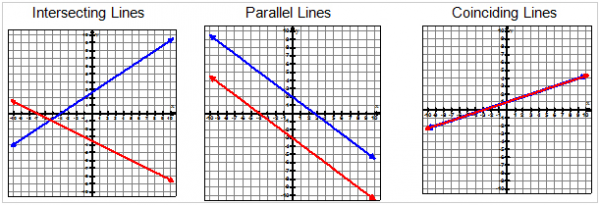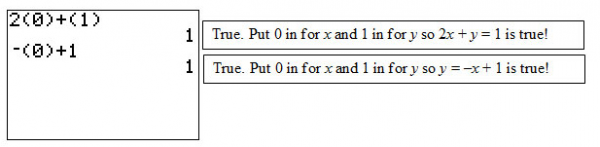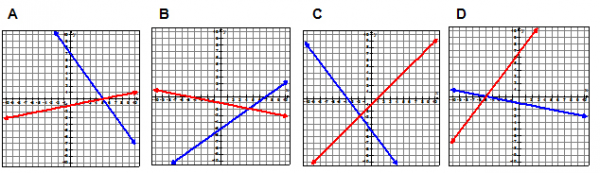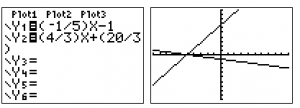# Let's Get Started

Let's explore how to graphically determine the solution to a system of linear equations. You will learn the different possible solutions scenarios and how to check that solution.

TEKS Standards and Student Expectations

A(3) Linear functions, equations, and inequalities. The student applies the mathematical process standards when using graphs of linear functions, key features, and related transformations to represent in multiple ways and solve, with and without technology, equations, inequalities, and systems of equations. The student is expected to:

A(3)(F) graph systems of two linear equations in two variables on the coordinate plane and determine the solutions if they exist

A(3)(G) estimate graphically the solutions to systems of two linear equations with two variables in real-world problems

Resource Objective(s)

Graphically determine the solution to a system of linear equations.

Essential Questions

How many solutions can you have to a systems of linear equations, and what are they?

Graphically, what do the solutions to a system of linear equations look like?

How can you check to see if your solution to your system is correct?

Vocabulary

# Solutions for Systems of Linear Equations

We're going to find solutions to systems of linear equations by looking at the graphs of the equations. A system of linear equations is a set of 2 or more linear equations with 2 or more of the same variables.

There are three possible solutions and situations for a system of 2 linear equations.

1. Intersecting Lines - One Solution

If the lines are intersecting lines, the solution is the coordinate point where the lines meet.

2. Parallel Lines - No Solution

Parallel lines do not cross, and there is no solution.

3. Coinciding Lines - Infinite Solutions

When the lines are the exact same, they are called coinciding lines and have infinite solutions because any point on one is also on the other line.

The following are the corresponding graphs of the different solutions.# An Applet for Linear Systems

It is helpful to use an interactive applet to understand what it means to solve a system of equations by graphing.

Before working with the applet, let's make sure we understand the information you are given on the screen.The equations in this applet are in slope intercept form (y = mx + b), the same form used on your graphing calculator. The first equation is the pink line, and the second equation is the blue line.

The slopes for the lines are in the upper right-hand corner. The slope for the pink line is a, and the slope for the blue line is s. Move the sliders a and s to observe the change in the slope.

The b in y = mx + b, or the y-intercept, are b and c respectively.

The solution is the point of intersection.

Practice by solving the system, $y=35x+1x-2y=-3$

The first equation is the pink line.

1. Move a to 3/5 (0.6)

2. Move b to 1

The second equation is the blue line.

1. First re-write the equation from standard form to slope-intercept form by solving for y. You should get: = 1/2x + 1.5

2. Move s to 1/2 (0.5)

3. Move c to 1.5

The solution is the point of intersection. Find the solution.

Now you are going to use the applet to solve each system of equations.Solving Systems of Linear Equations

Any solution to a system of equations can be checked, and it's very important that you check your answers.

To check a solution to a system, substitute the x- and y-coordinates for both equations.

Example

If (0, 1) is the solution to the system of equations $2x+y=1y=-x+1$, then substituting (0, 1) into BOTH equations ought to result in two true statements.# Systems of Parallel Lines

What happens if the lines are parallel? What is the solution to the system of equations?

Put the following pairs of slope-intercept equations into the applet and see what happens.

1. y = -2x + 4 and y = -2x - 3
2. y = 3x + 1 and y = 3x - 3
3. y = 0.5and y = 0.5+ 2Solving Systems of Linear Equations

# Systems of Coinciding Lines

What happens if the lines are on top of each other (coinciding)? What is the solution to the system of equations?

Put the following pairs of equations into the applet and see what happens.

1. 4x + 2y = 8 and 2x + y = 4
2. x - 2y = 6 and y = 0.5x + 3

Remember that you may need to convert your equation into slope-intercept form before you enter it into the interactive applet.Solving Systems of Linear Equations

# Calculator Practice

How can you use your calculator to determine which of the following graphs best represents the solution to the following system of equations $x+5y=-54x-3y=-20$?To use the calculator to answer this question, the equations need to be in slope-intercept form. To convert, solve each equation for y.

Using these equations, plug them in for the "Y1" and "Y2" values in your graphing calculator. Graph them, and match the correct answer choice.The graph from the calculator most looks like graph D because it crosses on the x-axis between the 2nd and 3rd quadrants. None of the other graphs do.

Is there a way to check your work?

How can you use the slope-intercept equations to check your work? Perhaps you could double check the y-intercept of the lines and/or the slopes.

Where do the two lines intersect? You can use the intersection function under the [CALC] menu to find the intersection.